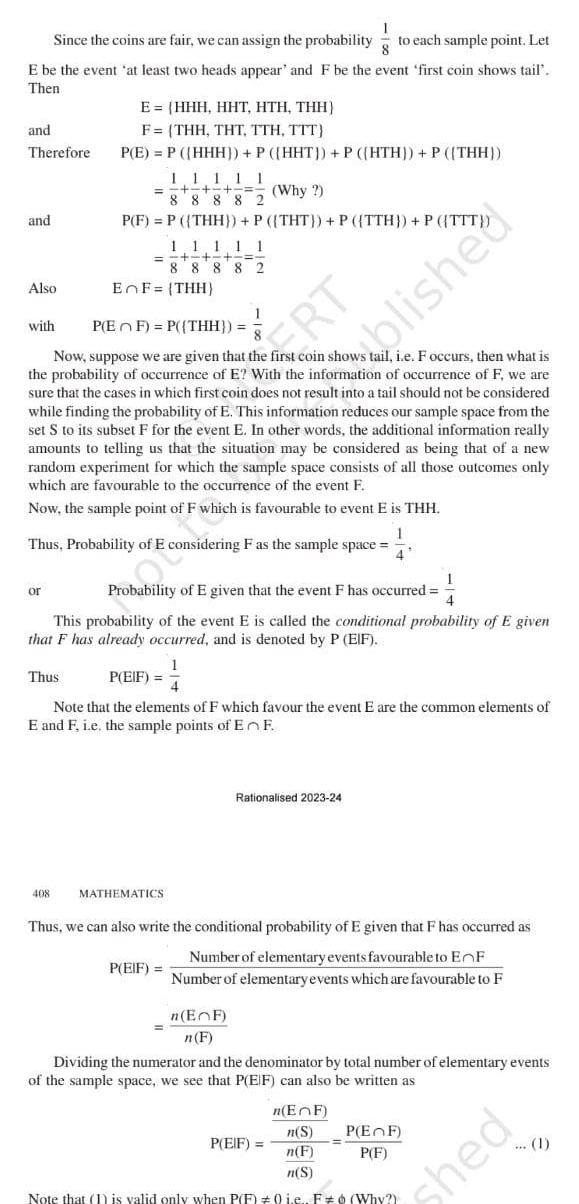Question:

# E be the event at least two heads appear and F be the event

Last updated: 9/19/2023E be the event at least two heads appear and F be the event first coin shows tail Then and Therefore and 1 Since the coins are fair we can assign the probability to each sample point Let Also with or E HHH HHT HTH THH F THH THT TTH TTT P E P HHH P HHT P HTH P THH Thus 1 1 111 Why 88 882 P F P THH P THT P TTH P TTT 408 11111 8 8 8 8 2 P EF P THH Now suppose we are given that the first coin shows tail i e F occurs then what is the probability of occurrence of E With the information of occurrence of F we are sure that the cases in which first coin does not result into a tail should not be considered while finding the probability of E This information reduces our sample space from the set S to its subset F for the event E In other words the additional information really amounts to telling us that the situation may be considered as being that of a new random experiment for which the sample space consists of all those outcomes only which are favourable to the occurrence of the event F Now the sample point of F which is favourable to event E is THH 1 Thus Probability of E considering F as the sample space 4 EnF THH 1 Probability of E given that the event F has occurred 4 This probability of the event E is called the conditional probability of E given that F has already occurred and is denoted by P EIF P EIF MATHEMATICS Note that the elements of F which favour the event E are the common elements of E and F i e the sample points of En F RT 1 P EIF Thus we can also write the conditional probability of E given that F has occurred as Number of elementary events favourable to EnF Number of elementary events which are favourable to F n EF n F Rationalised 2023 24 blished Dividing the numerator and the denominator by total number of elementary events of the sample space we see that P EIF can also be written as n EnF n S n F n S Note that 1 is valid only when P F 0 i e F Why P EIF P EF P F hed 1